# SPICE modeling of Magnetic Core from Datasheet

we can set two geometrical parameters for the support ETD49, reporting the AREA and PATH parameters from the datasheet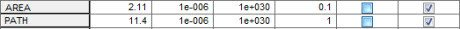From the N67 ferrite datasheet we find a value of Iniatial permeability of 2100.Write this data in the edit field: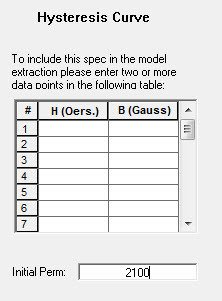Now we have to report the couple (H,B) of points delineating the Hysteresis curve from datasheet: recall that

1 Tesla = 10000 Gauss, and 1 Oersted = 79.577 A/m

Before insert the points, let’s set the X rage axis from -20 to 20 Oersted for example, and the Y axis to -5000 to 5000 Gauss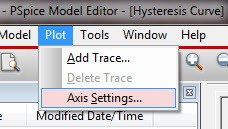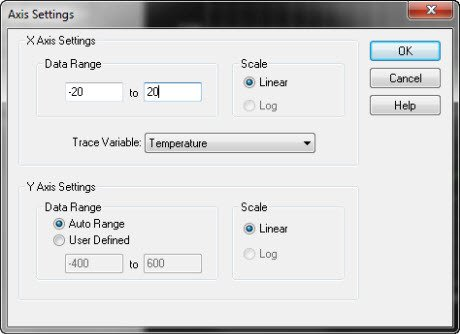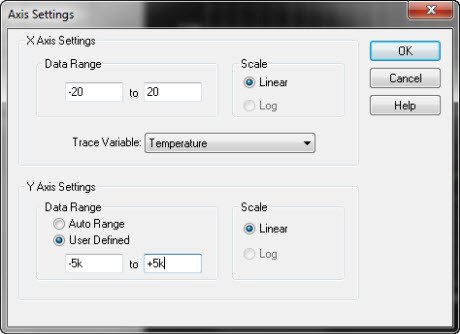From the Epcos datasheet  derive a graph useful for tracing the dynamic magnetization curve through couple of points B-H: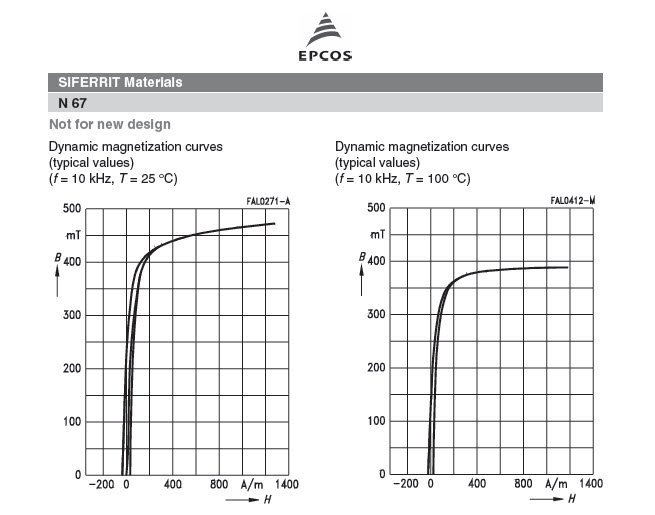despite of what we could think, report a lot of points could make the interpolation algorithm provide worst results, in this example we report 12 couples of points along the curve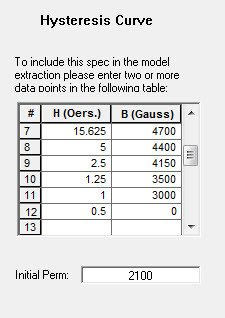click on the extract icon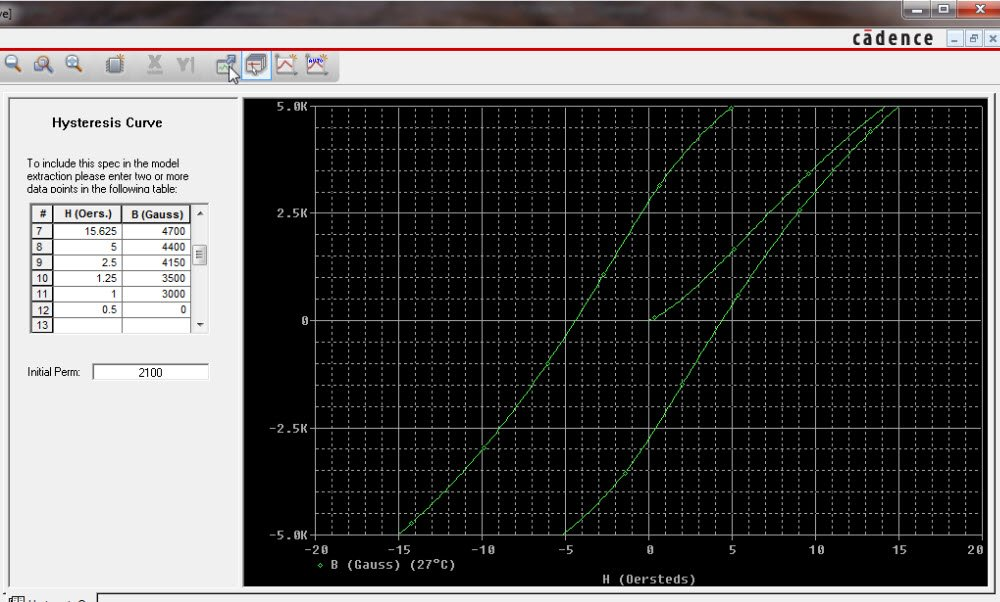below the resultant Hysteresis curve:the parameters window shows the calculated dataOur Model of Core ETD49 N67 will be: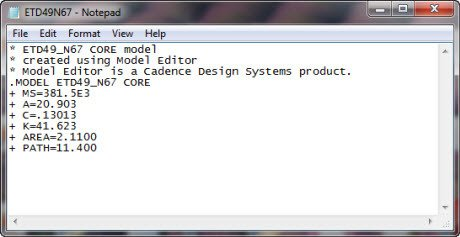Note that this model core hasn’ t the air gap.